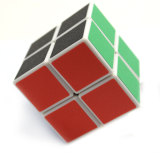# $\mbox{R}_2\mbox{U}_2$ Back To The Future

Probability Level 2Consider the $2\times 2\times 2$ cube puzzle, shown above. It consists of 8 pieces that start out in the solved orientation and can be transformed into alternative orientations by rotating any of the six faces.

To start, let us consider the two moves $\mbox{R}_2$ and $\mbox{U}_2$. When $\mbox{R}_2$ is performed, the right side of the cube is spun two quarter rotations clockwise. Similarly, $\mbox{U}_2$ indicates that the top layer ($\mbox{U}=$ Up) is spun two quarter rotations clockwise. Whenever a face gets two quarter turns, each piece in that face ends up diagonal to its original position on the face.

We start transforming the cube by performing $\mbox{R}_2$ followed by $\mbox{U}_2$. We call this sequence of events the $\mbox{R}_2\mbox{U}_2$ permutation. How many $\mbox{R}_2\mbox{U}_2$ permutations do we go through before the $2\times 2\times 2$ cube is back to its original state?

Note: The $\mbox{R}_2$ and $\mbox{U}_2$ moves are displayed below on a $3\times3\times3$ cube.

• $\mbox{R}_2$• $\mbox{U}_2$×